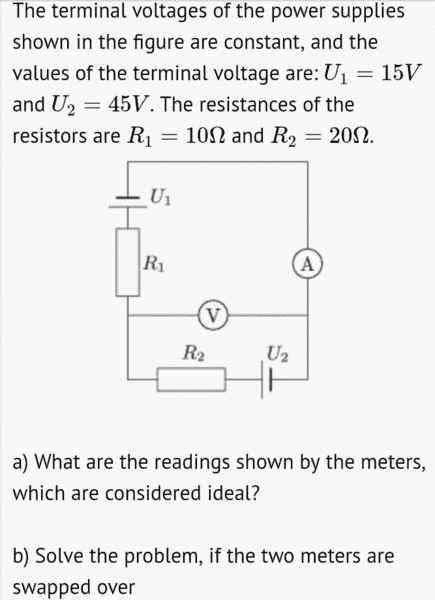# Reading of a voltmeter and an ammeter

• Jahnavi
In summary: This means that they will only read a very small current and may not be able to measure a potential difference at all.

## Homework Statement## The Attempt at a Solution

Part a)

Voltmeter can be replaced by an open circuit and ammeter can be replaced by a short circuit .By doing so the current flows in the outer loop consisting of both the batteries and both the resisters .The current in the circuit is given by i = (45-15)/(20+10) = 1A .

Potential drop across Voltmeter is U2-iR2 = 45-(1)(20) = 25 V

So reading of ammeter is 1A and that of voltmeter is 25V .

Is that the correct answer for part a) ?

I am having some trouble with the use of "ideal voltmeter" .An ideal voltmeter has infinite resistance .It can be replaced by an open circuit . But if voltmeter has to read a potential difference it must draw some current .If no current flows through the voltmeter ,then it's reading would be simply zero .

Sorry for being a bit confused .

#### Attachments

Jahnavi said:
Is that the correct answer for part a) ?
yes

I am having some trouble with the use of "ideal voltmeter" .An ideal voltmeter has infinite resistance .It can be replaced by an open circuit . But if voltmeter has to read a potential difference it must draw some current .If no current flows through the voltmeter ,then it's reading would be simply zero .
Your analysis is correct, BUT ... ideal volt meters are assumed to draw an infinitesimally small current that has zero effect on the circuit. It's a problem-simplification technique.

•Jahnavi
OK

And in part b) when the two meters are interchanged

No current flows through U1 and R1 , so entire terminal voltage of U1 appears across the voltmeter .It reads 15V .

Current flows in the loop consisting ammeter and U2 and R2 .Current i = 45/20 = 2.25A .

I hope this part is also correct .

Jahnavi said:
I hope this part is also correc
Yes.

•Jahnavi
Just for info... Some specialist Voltmeters have very high input resistance, as much as 10*109 Ohms on some ranges.